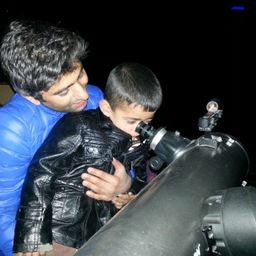## a) 65.22 beats/minb) 11 beats/min

Gravitation

### Discussion

You must be signed in to discuss.
##### Top Physics 101 Mechanics Educators##### Christina K.

Rutgers, The State University of New Jersey##### Andy C.

University of Michigan - Ann ArborLectures

Join Bootcamp

### Video Transcript

Well, speed of astronaut relative to earth is equal to zero point 500 times the speed of light. This is the speed off astronaut relative, uh, to art and boss. Raid of astronaut is also given no balls. Great off astronaut Astronaut is equal to 7 75 beats permanent 75 beats for a minute. All right apart again using ah, Delta T is equal to Delta TP, which is proper time divided by squared Rudolph one minus we squared, divided by C square on DDE Proper time is one minute to be tickles 60 seconds divided by squared Will door one minus Well, we is Ah, a 0.500 time to the speed of light all squared, divided by C square And this equals 60 seconds divided by squared Rudolph one minus zero points to 5.25 and therefore Delta T Delta T is equal to 60 divided by zero point 0.8 66 and this gives us 1.15 minutes 1.1 five minute. So don't It is equal to 1.15 minute. Uh, so pulse rate major by, uh, the Earth hopes ever 12th Street major by the Earth Observer Both rate majored, measured by the Earth observer by the Earth Hopes ever Observer is Ah, 75 divided by 1.15 and this gives us 65 point ah to two beats per minute. Our minute. Now let's to part B What would be the ball straight if, uh, re is a cool too zero point mine nine zero times the speed of light. So again, using Delta T is equal to Delta t p divided by scared Rudolph one minus we square divided by C square. Um, appropriate time is 60 seconds divided by square Rudolph one minus. We in these cases, uh, zero point mine 90 and we have scratched. C square gets cancelled C square and therefore we have 60 divided by, uh, 0.140 point 14 and 60. Divided by 0.14 is equal to, uh, Fuller to eight point 57 seconds. 57 seconds and minutes. Delta T is equal to seven point 14 minutes and therefore all straight. Both rate is equal to 75 divided by 7.14 which gives us 10.50 o r Ah beat, which is approximately cool to 11 beats per minute are I mean it.#### Topics

Gravitation

##### Top Physics 101 Mechanics Educators##### Christina K.

Rutgers, The State University of New Jersey##### Andy C.

University of Michigan - Ann ArborLectures

Join Bootcamp